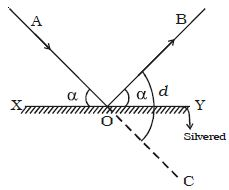# Explain Deviation of Light by Plain Mirror

Deviation of Light by Plain Mirror: Consider a ray of light, AO, incident on a plane mirror XY (Figure) at O. It is reflected along OB. The angle AOX made by AO with XY is known as the glancing angle α with the mirror.Since the angle of reflection is equal to the angle of incidence, the glancing angle BOY made by the reflected ray OB with the mirror is also equal to α. The light has been deviated from a direction AO to α direction OB. Since angle COY = angle AOX, it follows that angle of deviation, d = 2α

So, in general, the angle of deviation of a ray by a plane mirror or a plane surface is twice the glancing angle.